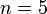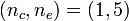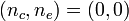# User:Tohline/Appendix/Ramblings

(Difference between revisions)
 Revision as of 16:41, 20 December 2016 (view source)Tohline (Talk | contribs) (→Mathematics)← Older edit Revision as of 16:45, 20 December 2016 (view source)Tohline (Talk | contribs) (→Mathematics)Newer edit → Line 54: Line 54:
• [[User:Tohline/SSC/Structure/Polytropes#CubicRoot|Srivastava's F-Type solution]] for [itex]~n=5[/itex] polytropes.
• [[User:Tohline/SSC/Structure/Polytropes#CubicRoot|Srivastava's F-Type solution]] for [itex]~n=5[/itex] polytropes.
• [[User:Tohline/SSC/Structure/BiPolytropes/Analytic1_5#Analytic_Solution_of_Key_Interface_Relation|Murphy & Fiedler's Bipolytrope]] with [itex]~(n_c, n_e) = (1,5)[/itex]
• [[User:Tohline/SSC/Structure/BiPolytropes/Analytic1_5#Analytic_Solution_of_Key_Interface_Relation|Murphy & Fiedler's Bipolytrope]] with [itex]~(n_c, n_e) = (1,5)[/itex]
• +
• [[User:Tohline/Appendix/Ramblings/Additional_Analytically_Specified_Eigenvectors_for_Zero-Zero_Bipolytropes#Solve_Cubic_Equation|Analytic Eigenfunctions for Bipolytropes]] with [itex]~(n_c, n_e) = (0, 0)[/itex]
• Roots of Quartic Equation
• Roots of Quartic Equation

## Ramblings

Sometimes I explore some ideas to a sufficient depth that it seems worthwhile for me to archive the technical derivations even if the idea itself does not immediately produce a publishable result. This page, which has a simple outline layout, provides links to these various pages of technical notes.

1. Toroidal configurations & related coordinate systems
2. Relationship between HNM82 models and T1 coordinates
3. Orthogonal Curvilinear Coordinates
4. Playing with the Spherical Wave Equation
5. Analyzing Azimuthal Distortions
6. Integrals of Motion
1. Old discussion
2. T3 Coordinates
1. Special (quadratic) case: Joel's Derivation vs. Jay's Derivation
3. Killing Vector Approach; Jay Call's related Talk page
4. Characteristic Vector for T3 Coordinates
5. T4 Coordinates (Abandoned by Joel 7/6/2010 because non-orthogonal)
8. Photosphere of Stably Accreting DWD
9. Initial Effort to Explain Jay Call's Hybrid Scheme in the Context of Zach Byerly's Dissertation
10. Exploring the Properties of Radial Oscillations in Pressure-Truncated n = 5 Polytropes
11. Instabilities Associated with Equilibrium Sequence Turning Points
12. Searching for Additional Eigenvectors for Zero-Zero Bipolytropes
13. Investigation Resulting from a July, 2013 Discussion with Kundan Kadam

## Mathematics

1. Roots of Cubic Equation
1. PP Tori — Also includes cube root of a complex number
2. Srivastava's F-Type solution for$~n=5$ polytropes.
3. Murphy & Fiedler's Bipolytrope with$~(n_c, n_e) = (1,5)$
4. Analytic Eigenfunctions for Bipolytropes with$~(n_c, n_e) = (0, 0)$
2. Roots of Quartic Equation
1. Analytic Eigenfunction for Bipolytropes with$~(n_c, n_e) = (0, 0)$
2. Determine temperature from total pressure
3. Singular Sturm-Liouville (eigenvalue) Problem
1. Oscillations of PP Tori in the slim torus limit
2. Characteristics of unstable eigenvectors in self-gravitating tori

 © 2014 - 2021 by Joel E. Tohline |   H_Book Home   |   YouTube   | Appendices: | Equations | Variables | References | Ramblings | Images | myphys.lsu | ADS | Recommended citation:   Tohline, Joel E. (2021), The Structure, Stability, & Dynamics of Self-Gravitating Fluids, a (MediaWiki-based) Vistrails.org publication, https://www.vistrails.org/index.php/User:Tohline/citation